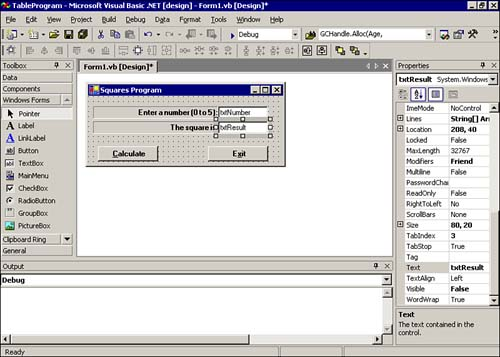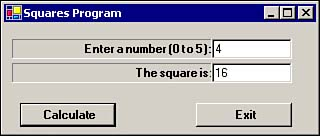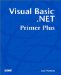# Multidimensional Arrays

As you have seen, an array is great for holding a list of data. The list of data might be the names of friends or things needed from the grocery store. Other situations, however, require that you have a table of data. A data table is multidimensional. That is, tables of data have two dimensions: rows and columns . For example, suppose you wanted to have a table of squares for the integer values through 5 . You might write that table on a piece of paper like this:

Number

Square

1

1

2

4

3

9

4

16

5

25

In this example, the table has six rows and two columns. You can reconstruct this table with a simple program.

You should start a new project and call it TableProgram . Then, you need to place two labels, two text boxes, and two buttons on the form, as shown in Figure 7.4. As shown in Figure 7.4, you should name the Calculate button btnCalc and the Exit button btnExit . As in the days-of-the-week program, you need to set the Visible property of the txtResult text box and its associated label ( lblResult ) to False so that they are invisible until after the Calculate button is clicked.

##### Figure 7.4. Component placement for the TableProgram program.Now you need to add the following code to the btnCalc object's Click() event:

` Dim SquaresTable(5, 1) As Integer  SquaresTable(0, 0) = 0 SquaresTable(0, 1) = 0 SquaresTable(1, 0) = 1 SquaresTable(1, 1) = 1 SquaresTable(2, 0) = 2 SquaresTable(2, 1) = 4 SquaresTable(3, 0) = 3 SquaresTable(3, 1) = 9 SquaresTable(4, 0) = 4 SquaresTable(4, 1) = 16 SquaresTable(5, 0) = 5 SquaresTable(5, 1) = 25 txtResult.Text = CStr(SquaresTable(CInt(txtNumber.Text), 1)) lblResult.Visible = True txtResult.Visible = True `

You should look at the dimension statement for the SquareTable() array. The Dim statement tells Visual Basic .NET to define an array that has through 5 rows and through 1 columns. This array layout is shown in Table 7.1.

##### Table 7.1. Indexes for the SquaresTable() Array

N

Square of N

0, 0

0, 1

1, 0

1, 1

2, 0

2, 1

3, 0

3, 1

4, 0

4, 1

5, 0

5, 1

The next 12 program statements fill in the actual values for the array. These 12 program statements result in a table that looks like Table 7.2.

##### Table 7.2. Values in the SquaresTable() Array

N

Square of N

1

1

2

4

3

9

4

`  16  `

5

`  25  `

This statement:

` txtResult.Text = CStr(SquaresTable(CInt(txtNumber.Text), 1)) `

simply converts the value typed in by the user into an integer value that is to be used as the index into the array. For example, if the user types 4 into the txtNumber text box, the statement is processed as follows :

` txtResult.Text = CStr(SquaresTable(CInt(txtNumber.Text), 1))  txtResult.Text = CStr(SquaresTable(CInt("4"), 1)) txtResult.Text = CStr(SquaresTable(4, 1)) txtResult.Text = CStr(16)    ' Position 4,1 in Table 7.2 is 16 txtResult.Text = "16"    ' Made into a string for text box `

The string "16" is placed in the txtResult text box. The remaining two lines simply make the lblResult and txtResult controls visible. A sample run of this program is shown in Figure 7.5.

##### Figure 7.5. A sample run of the TableProgram program.You should walk through each line of code shown in Listing 7.1 and confirm that the program displays the proper result:

##### Listing 7.1 Complete Code for the TableProgram Program
` Public Class frmTable  Inherits System.Windows.Forms.Form  Private Sub btnCalc_Click(ByVal sender As System.Object, ByVal e As System.EventArgs) Handles btnCalc.Click   Dim SquaresTable(5, 1) As Integer   Dim MyArray(,) As Integer   '  ReDim MyArray(10, 10)   ReDim MyArray(3, 5)   SquaresTable(0, 0) = 0   SquaresTable(0, 1) = 0   SquaresTable(1, 0) = 1   SquaresTable(1, 1) = 1   SquaresTable(2, 0) = 2   SquaresTable(2, 1) = 4   SquaresTable(3, 0) = 3   SquaresTable(3, 1) = 9   SquaresTable(4, 0) = 4   SquaresTable(4, 1) = 16   SquaresTable(5, 0) = 5   SquaresTable(5, 1) = 25   txtResult.Text = CStr(SqaresTable(CInt(txtNumber.Text), 1))   lblResult.Visible = True   txtResult.Visible = True  End Sub  Private Sub btnExit_Click(ByVal sender As System.Object, ByVal e As System.EventArgs) Handles btnExit.Click   Me.Dispose()  End Sub End Class `Visual Basic .NET Primer Plus
ISBN: 0672324857
EAN: 2147483647
Year: 2003
Pages: 238
Authors: Jack Purdum

Similar book on Amazon